# Construct NFA with Epsilon moves for regular expression a+ba*.

The regular expression R= a+ba* divided into r1 and r2

r1= a and r2= ba*

Let us draw Non-deterministic finite automata (NFA) for r1 as given below −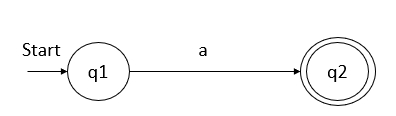Now, we will go for r2 = ba *

Divide r2 into r3 and r4, where, r3=b and r4=a*

The NFA for r3 is as follows −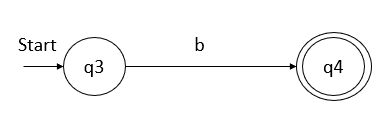The NFA for r4 is as follows −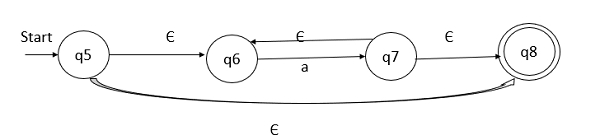q5 on epsilon movies goes to q6 and q8, q6 on ‘a’ goes to q7 whereas, q7 on epsilon moves goes to q6 as well as q7.

r2= r3.r4

Now, concatenate r3 and r4 as shown below −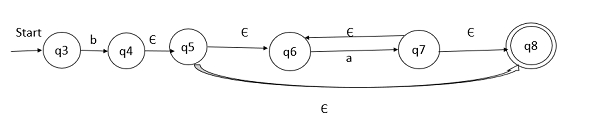q3 on input ‘b’ goes to q4, q4 on epsilon moves goes to q5, q5 on epsilon moves goes to q6, q6 on input ‘a’ goes to q7, whereas q7 on epsilon moves goes to q6 and q8.

Now, combine all expressions i.e. R=r1+r2 =a+ba* as shown below −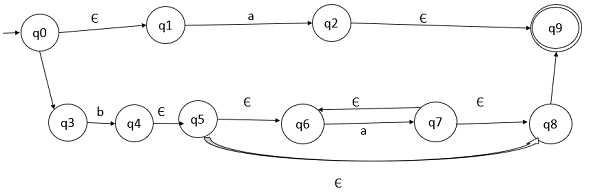The above diagram is the final NFA with moves for the given regular expression a+ba*.